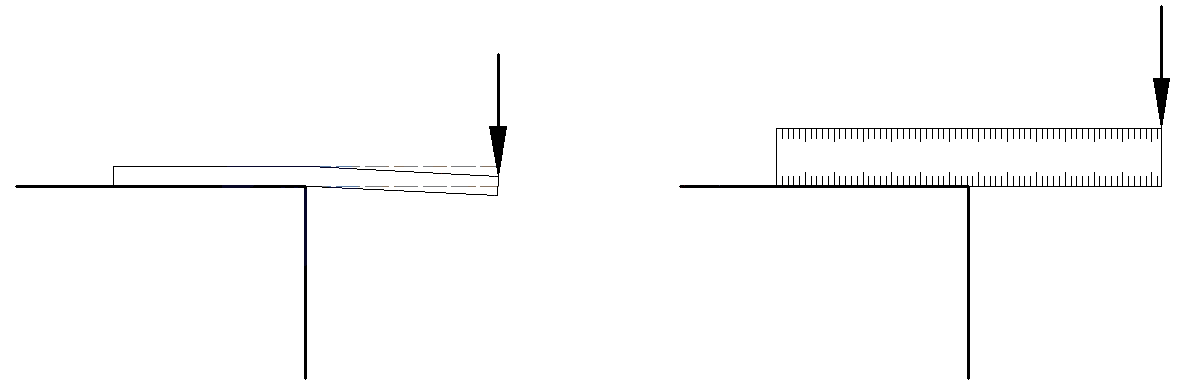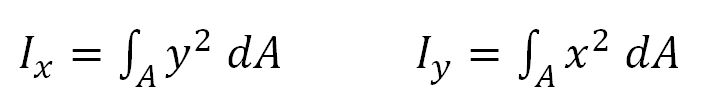# Glossary of Terms - Second Moment of Area Explained

## What is second moment of area?

Also commonly referred to as the Area Moment of Inertia, this geometric property of area distribution having a unit value of length to the fourth power (L4) can be defined as:

# For the Layman:

“A geometric property used to describe the effect a shape has on the stiffness or resistance to bending.”

To help visualise this geometric property think of a 30cm plastic ruler, similar to one you probably used in school. Imagine placing the ruler flat on a table and sliding the ruler so that half of the ruler is over hanging the table edge. Now, holding the ruler on the table firmly and pushing on the free end of the ruler it can be seen that with minimal force the ruler will bend slightly. If however, the ruler is turned on its edge (see diagram below) the ruler will not bend as easily. This property which depends on shape and often orientation is described by the second moment of area.# For the Engineer:

“The sum of all elementary products of area elements and the squares of their respective distances from the centroidal axis.” Or...Where Ix and Iy are the Second Moments of Areas of an area A about their respective axis where dA is the area under consideration, y is equal to the distance from the area to the x axis and x is the distance from the area to the y axis.

Values for various second moment of areas or moment of inertias can be found by selecting the shape under consideration in Geometric Properties.